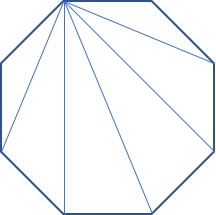finding the number of diagonals in a polygon

How many diagonals are there in an octagon?Instead of trying to count them all, use the formula for the number of diagonals in any polygon:$d= \dfrac{n(n-3)}{2}$

where n = the number of sides and d= the number of diagonals. For an octagon, n= 8, so$d= \dfrac{8(8-3)}{2} = 20$

This is a good formula to memorize and its derivation is pretty simple, so let’s see it. We will use the same octagon diagram, but only draw the diagonals from one vertex:You can see that from this vertex on the upper left, a diagonal can be drawn to every vertex except the two adjacent vertices and to itself. So the number of diagonals from any vertex in an n-gon is (n-3). Since there are n vertices, the total number of diagonals is n(n-3). But we’ve just counted every diagonal twice (once from each endpoint), so to find the number of different diagonals, we divide by two. This gives us the formula above.

Geometry
Blue Taste Theme created by Jabox